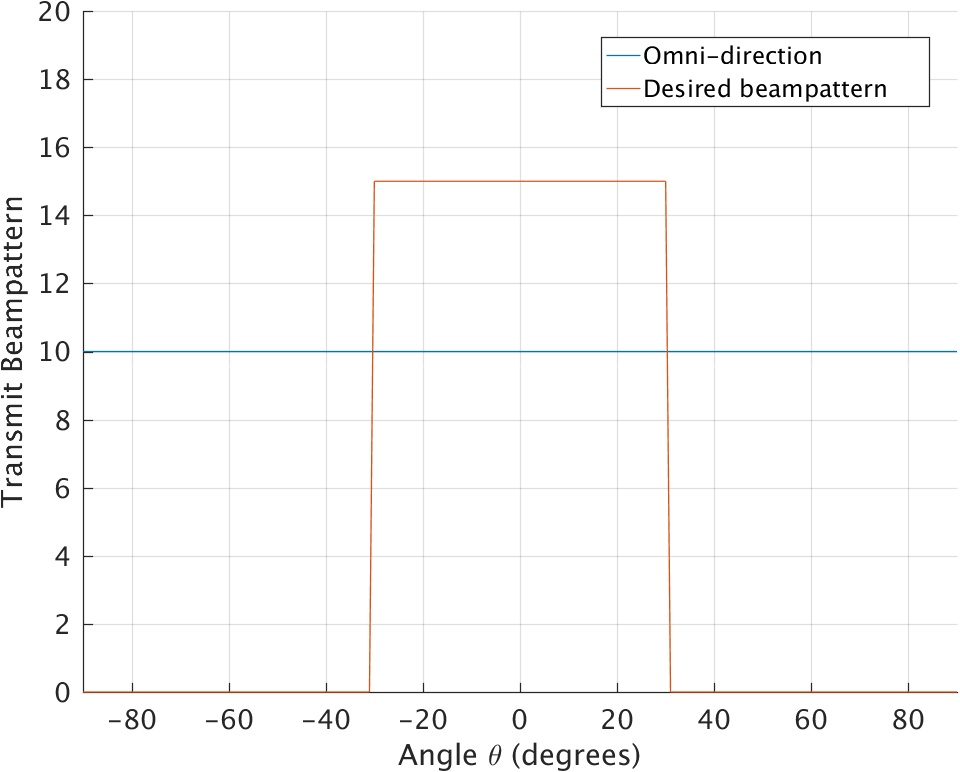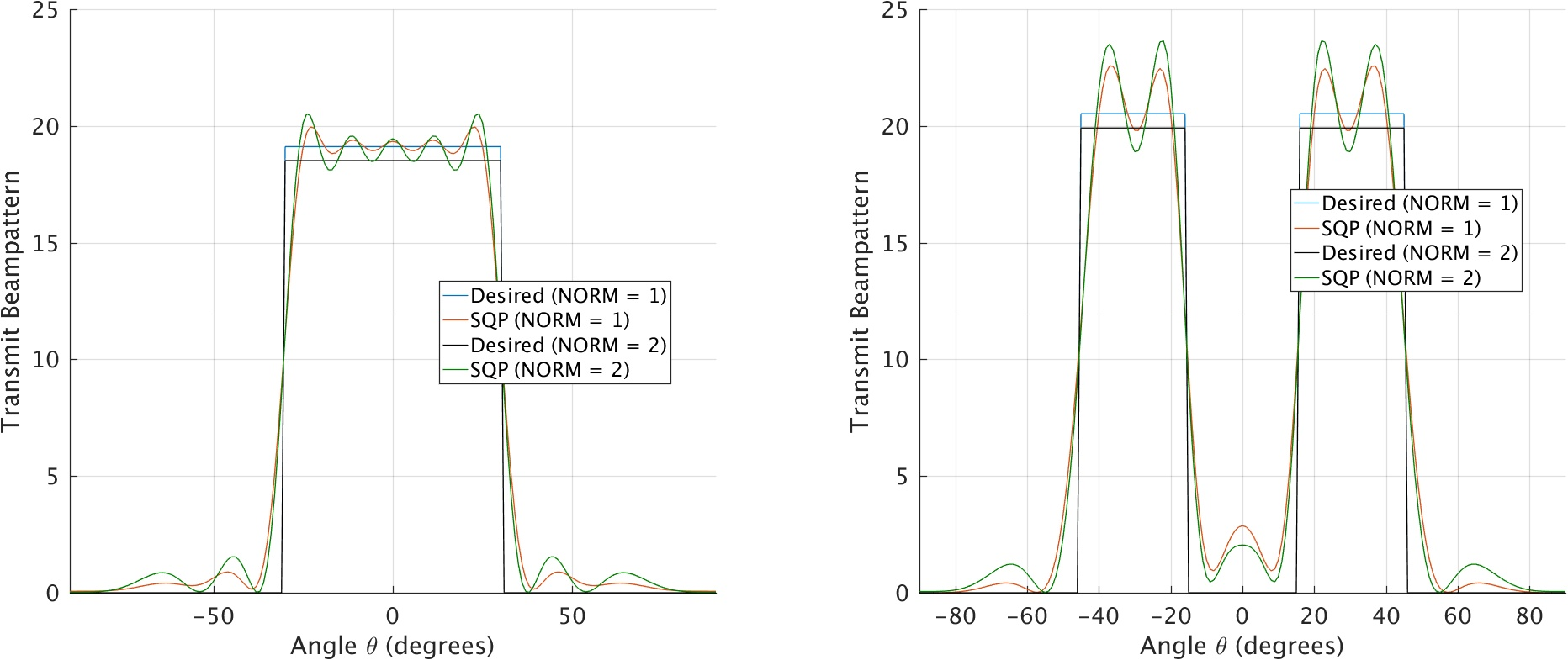# Beamforming

Single antennas are commonly omni-directional, which means the transmitted power is distributed identically in all directions. In order to maximize the transmitted power in a region of interest (ROI) and minimize it in all other directions, some sort of beamforming is required. The radiation pattern can be altered mechanically using a waveguide (i.e. horn antenna), or by changing the antenna shape (i.e. micro-strip antennas). However, these methods generate a permanent beampattern that cannot be changed depending on the use-case.

For this reason, electronic beamforming or beampattern matching attracted a lot of attention. This signal processing technique uses an array of omni-directional antennas to transmit carefully designed signals and obtain a constructive interference in the ROI and destructive interference elsewhere. It consists in designing waveforms with specific cross-correlation properties to approach a desired beampattern.Desired beampattern with ROI=[-30°, 30°]. Thanks to the constructive interference, the received power in the ROI increases while the transmitted power remains the same.

To achieve this, a uniform linear array of $N$ antenna elements with half wavelength inter-element spacing is used. Let $x_n(l)$ be the baseband signal transmitted from antenna $n$ at time index $l$. The received baseband signal at location $\theta_k$ is defined as $$r_k(l)=\sum_{n=1}^N \mathrm{e}^{-j (n-1) \pi \sin(\theta_k)}x_n(l),\quad l=1,2,\ldots ,L,$$ where $L$ denotes the total number of symbols transmitted from each antenna. By defining the vectors ${\bf e}(\theta_k) = [1 \quad \mathrm{e}^{j \pi \sin(\theta_k)} \quad \ldots \quad \mathrm{e}^{j(N-1)\pi \sin(\theta_k)}]^T$ and ${\bf x}(l) = [x_1(l) \quad x_2(l) \quad \ldots \quad x_N(l)]^T$, we can simplify the above expression and write it in a following vector form
$$r_k(l)={\bf e}^H(\theta_k) {\bf x}(l).$$

Thus, the received power at location $\theta_k$ is expressed as follows
$$P(\theta_k) = E\left\{{\bf e}^H(\theta_k) {\bf x}(l) {\bf x}^H(l) {\bf e}(\theta_k) \right\} = {\bf e}^H(\theta_k) {\bf R} {\bf e}(\theta_k),$$ where $\bf R$ is the correlation matrix of the transmitted waveforms. To achieve the desired beampattern $\phi(\theta)$, the covariance matrix $\bf R$ should minimize the following constrained problem
$$\begin{eqnarray} \underset{{\bf R},\alpha}{\min}&\quad&\frac{1}{K} \sum_{k=1}^K\left|{\bf e}^H(\theta_k) {\bf R} {\bf e}(\theta_k)-\alpha \phi (\theta_k)\right|^L,\\ \text{subject to}& &{\bf v}^H {\bf R} {\bf v} \geq 0, \quad \text{for all }{\bf v}\\ && {\bf R}(n,n) = c, \quad \text{for n=1,2,\ldots, N, } \end{eqnarray}$$ where $K$ is the number of subdivisions of the region of interest,$\alpha$ is a weighting factor and $c$ is the transmitted power from each antenna. Here, $L\in \{1,2\}$ indicates if the problem optimizes the L-1 or L-2 norm. As $\bf R$ should be a positive covariance matrix, the first constraint is straightforward. Besides, the second constraint should be satisfied to achieve maximum power efficiency. Moreover, depending on the parity of the desired beampattern $\phi(\theta)$, the designed covariance matrix $\bf R$ can contain real or complex elements.Designed beampattern in case the region of interest is [-30°, 30°] $$left$$ or [-45°, -15°]U [15°,45°] $$right$$. The number of antenna elements is N=10 and each antenna transmits a unit power (c=1).

From the figure above, it can be noticed that the solution of the L-1 optimization problem performs better than the L-2 norm. In fact, it has lower ripples in the region of interest, has lower side lobes and achieves higher transmitted power. Conversely, this gain comes at the expense of higher computational complexity to determine the L-1 norm solution.

In some cases, it is also important to minimize the cross-correlation beampattern term ${\bf e}^H(\theta_l) {\bf R} {\bf e}(\theta_k)~(\text{for } \theta_l \neq \theta_k)$. Thus, the cost function becomes
$$\begin{eqnarray} \underset{{\bf R},\alpha}{\min}&\quad&\frac{1}{K} \sum_{k=1}^K\left|{\bf e}^H(\theta_k) {\bf R} {\bf e}(\theta_k)-\alpha \phi (\theta_k)\right|^L + \frac{2 \lambda}{K^2-K} \sum_{u=1}^{K-1} \sum_{v=u+1}^{K} \left|{\bf e}^H(\theta_u) {\bf R} {\bf e}(\theta_v)\right|^L, \end{eqnarray}$$ where $\lambda$ defines the weight of the second term.

The references below suggest a number of methods to solve the optimization problem. Once the covariance matrix $\bf R$ is determined, the next goal is to design practical waveforms that achieve specific cross-correlation properties.

1. D. R. Fuhrmann and J. S. Antonio, “Transmit beamforming for MIMO radar systems using partial signal correlation,” in Proc. 38th Asilomar Conf. Signals, Syst. Comput., Nov. 2004, vol. 1, pp. 295–299.
2. D. R. Fuhrmann and J. S. Antonio, “Transmit beamforming for MIMO radar systems using signal cross-correlation,” IEEE Trans. Aerosp. Electron. Syst., vol. 44, pp. 171–185, Jan. 2008.
3. T. Aittomaki and V. Koivunen, “Low complexity method for transmit beamforming in MIMO radar,” in Proc. IEEE Int. Conf. Acoust., Speech, Signal Process. (ICASSP), Apr. 2007, pp. 305–308.
4. T. Aittomaki and V. Koivunen, “Signal covariance-matrix optimisation for transmit beamforming in MIMO radars,” in Proc. 41st Asilomar Conf. Signals, Syst. Comput., Nov. 2007, pp. 182–186.
5. P. Stoica, J. Li, and Y. Xie, “On probing signal design for MIMO radar,” IEEE Transactions on Signal Processing, vol. 55, pp. 4151–4161, Aug. 2007.
6. S. Ahmed, J. S. Thompson, Y. R. Petillot and B. Mulgrew, “Unconstrained Synthesis of Covariance Matrix for MIMO Radar Transmit Beampattern,” in IEEE Transactions on Signal Processing, vol. 59, no. 8, pp. 3837-3849, Aug. 2011.